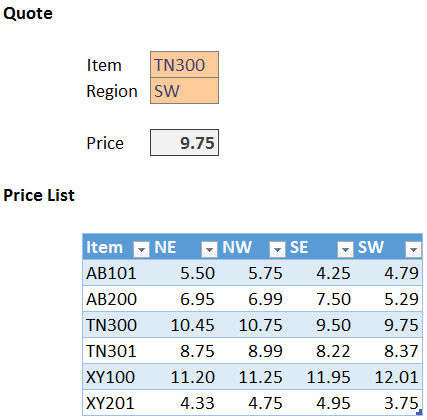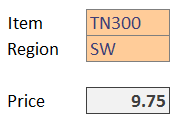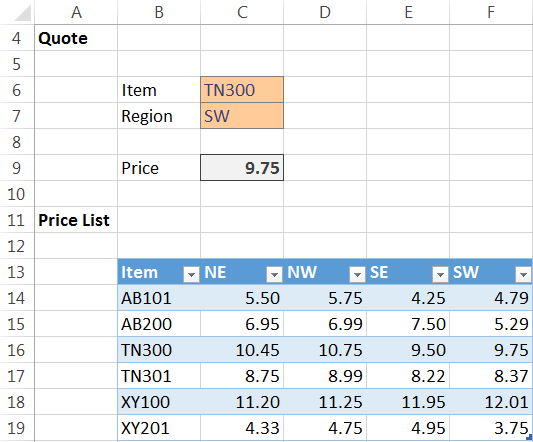# Two-Dimensional VLOOKUPIn this post, we’ll perform a two-dimensional lookup with Excel’s VLOOKUP function.

## Objective

Let’s begin by clarifying our objective and what is meant by the term two-dimensional lookup. We have stored our price list in a table, and the price for each item varies based on the region. This is illustrated in the screenshot below.To create a quote for a customer, we’ll enter the item and the region and we want Excel to retrieve the correct price, as illustrated below.This is considered a two-dimensional lookup because we are asking Excel to look down through the rows to find the correct item, and then right through the columns to find the correct region. Although VLOOKUP typically performs one-dimensional lookups, with a little hack we can convince it to do two-dimensional lookups. Ready? Me too!

## VLOOKUP

The VLOOKUP function has four arguments, and our little hack involves the third argument. Let’s take a quick look at the arguments.

`=VLOOKUP(lookup_value, table_array, col_index_num, [range_lookup])`

Where

• lookup_value is the value we are trying to find
• table_array is the lookup range
• col_index_num is the column that has the value to return
• [range_lookup] tells Excel if we are doing a range lookup

Typically, the col_index_num argument is expressed as an integer value, such as 2 for the second column or 5 for the fifth column. However, if we use a function to determine the column number instead, then, VLOOKUP is able to look down for a matching item AND right for a matching region. The function we need here is MATCH.

## MATCH

The MATCH function returns the relative position of a list item. It has the following three arguments.

`=MATCH(lookup_value, lookup_array, [match_type])`

Where

• lookup_value is the value we are trying to find
• lookup_array is the lookup range
• [match_type] will be zero for exact match

The idea of our little hack is to use the MATCH function to tell the VLOOKUP function which column stores the prices for the specified region.

Rather than enter an integer, such as 2, for the third VLOOKUP argument, we’ll use MATCH instead. For example, let’s revisit our price quote worksheet pictured below.The formula in C9 that retrieves the correct price from the tbl_price table based on the item and region is shown below.

`=VLOOKUP(C6,tbl_prices,MATCH(C7,tbl_prices[#Headers],0),0)`

Where

• C6 is the item value we are trying to find
• tbl_prices is the lookup range, the prices table
• MATCH(C7,tbl_prices[#Headers],0) returns the column number of the specified region
• Where
• C7 is the region value we are trying to find
• 0 means exact match
• 0 means exact match

Now as we change the item and region values, our hacked VLOOKUP function will retrieve the correct price from the table…and that is a two-dimensional lookup with VLOOKUP!

Also note that since this is Excel, there are of course other options. For example, we could use the INDEX/MATCH combination instead, and if you are interested in that, check out the previous blog post referenced below.

Get a quick email notice when a new Excel article is available

• This field is for validation purposes and should be left unchanged.1.Rick

Jeff,

Now aren’t you slick!!! I was trying to figure out a solution for this very issue a couple of weeks ago. I never thought to use MATCH instead of an integer. This is great!!! thanks for the tip. Almost finished with Excel University Will start Vol 3 soon. Still practicing Vol 2, which is kicking my hiney. Sometimes my cerebellum logic can get wrapped around Excel logic. Fun to learn though. Excel University is a great little course. Made my job soooo much easier.

1.jefflenning Post author

🙂

2.Larry Simpson

Retired CPA with small tax practice to keep me busy during winter months. I have used Excel for a number of years, but consider that I have only scratched the surface of what program can do.

3.Scott Davison

Jeff

I need some help with a relatively simple problem but for weeks I simply have not been able to get someone in a expert potion with Excel to give me some guidance, can you help.

My problem is: (I will try and explain as best I can what I need to do)

I have a quote creation template that in cell C4 allows you to select (from a drop down) an item/product from a long list of kits, with set descriptions. (These are mirrored exactly in the price list)

What I want to have is cell (F4) show a price based on the drop down item selected in C4, and the price based on the hire period shown in cell (G4) – Daily / Weekly / Monthly. The 2 parts are on the same worksheet, I have copied and pasted below the headings and columns references.

Quote Template
(C4) (F4) (G4)
Description Quote No: Item # Qty Unit Price Rate Base Total
Compressor, 175cfm @ 100 psi HQ-00004 1 6 60 Monthly 360

Price List:
(K4) (P4) (T4) (X4)
Equipment Models Daily Weekly Monthly
Compressor, 175cfm @ 100 psi Compare 143 717 2150

Big question:

How can I get Cell F4 to show a price that is matched to an item/product selected in C4 from a drop down, and the price is based then on one of 3 variables (daily, weekly or monthly) chosen again form a drop down in cell G4. All prices are in a table co-located on the same worksheet for ease of formula building.

If anyone can help me solve this I would genuinely appreciate it as I need to complete this prior to taking the next step. I am open to any suggestions.

Regards

Scott Davison
Qatar

1.jefflenning Post author

Scott,
Since the value you are trying to retrieve is a price, which is a numeric value, you can accomplish your goal with a SUMIFS function. I’ve written a blog post that shows how to perform a lookup on multiple conditions with SUMIFS here:

https://www.excel-university.com/vlookup-on-two-or-more-criteria-columns/

Hope it helps!
Thanks
Jeff

1.Scott Davison

Jeff

Thanks for the prompt reply, I will read through the blog and see how I can apply it and create a formula that will give me the price in the cell.

I am sure it will work, thanks again any other advice directly related to the question I have will be gratefully received.

I will be signing up for the excel uni course level 1 very soon.

Regards
Scott

1.jefflenning Post author

Excellent…best of luck…and look forward to seeing you in the LMS!

4.sam

How to solve this
We have given mobiles no. In one sheet
And second sheet we have mobile no, state, city, name.
Using we vlookup
In sheet one mobile no, we find two columns at one time. State and city. Using one formula v lookup

1.Kurt LeBlanc

Hey Sam,

Can you reword your question? I’m having trouble understanding it. Sorry for the misunderstanding!

Thanks,
Kurt LeBlanc

5.Jim Smith

Sheet2 cell A1=T01
Sheet2 cell A2=T02
Sheet2 cell A3=T03

On Sheet1 column A and B there are 7 rows; 4 of which start with T01, 2 of which start with T02, and 1 of which starts with T03
A1 B1
T01 This text1
T02 This text2
T03 This text3
T01 This text1
T01 This text1
T02 This text2
T01 This text1

I need to display on Sheet2 the following
A B C
T01 This text1 4
T02 This text2 2
T03 This text3 1

Is it possible to do this?

1.Kurt LeBlanc

Hey Jim

Yes you can:)

I recommend creating a unique list of the first column from sheet 1 then using SUMIFS to populate the other rows instead of a traditional lookup function because they will retrieve only 1 value. You want the aggregate of T01, T02, and T03, respectively. This blog will help you through the process of SUMIFS: https://www.excel-university.com/multiple-condition-summing-in-excel-with-sumifs/

Let me know if this helps!
Kurt LeBlanc

6.Jen
1.jefflenning Post author